Next: Equations of Motion Up: The Dynamics of Galaxies Previous: Simplifying Assumptions

# Hamilton's principle

Newton's laws of motion are often considered to be fundamental postulates for describing the motion of particles in a gravitational field, at least from our daily viewpoint. In a more general picture this is not so. Not only are they just a result of the general theory of relativity, they can also be derived from a more general principle, namely Hamilton's principle. Newton's laws of motion are just one example of equations that can be deduced from Hamilton's principle, the equations of motion for galaxies in an expanding Universe is another (see Section 3.3). The following description of this principle is mainly taken from Gold.

Hamilton's principle is an ``integral principle'', which means that it considers the entire motion of a system between time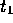and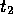. What is meant by this needs to be specified somewhat. The instantaneous configuration of the system is described by the values of n generalized coordinates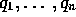, and corresponds to a particular point in a Cartesian hyperspace where the q-s form the n coordinate axes. This n-dimensional space is known as the configuration space. As the time evolves, the system point moves in this configuration space, tracing out a curve. This curve describes the path of motion of the system. The configuration space can be very different from the physical three-dimensional space, where only three coordinates are needed to describe a position at any give time. For example, a system that is being described both by the spatial coordinates and the velocities would have a six-dimensional configuration space at any given point in time.

Hamilton's principle is a version of the integral principle which considers the motion of a mechanical system, described by a scalar potential that may be a function of the coordinates, velocities and time. The integral, often also referred to as the action, is, in an essential one-dimensional form fromto, given by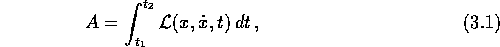where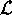is the Lagrangian, given by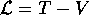, T and V being the kinetic and potential energy, respectively. The dot indicate derivative with respect to time. The dependence of x on t is not fixed; that is, x(t) is unknown. This means that although the integral is fromto, the exact path of integration is not known. The correct path of motion of the system is such that the action has a stationary value; i.e. the integral along the given path has the same value to within first-order infinitesimals as that along all neighbouring paths. The difference between two paths for a given t is called the variation of x,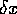, and is conventionally described by introducing a new function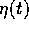to define the arbitrary deformation of the path and a scale factor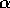to give the magnitude of the variation. The functionis arbitrary except for two restrictions: firstly, it must satisfy the boundary values,; secondly, it must be twice differentiable. The paths can then be described as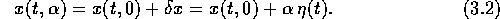We have a stationary value of the action when the derivative of A with respect to the scale factoris zero: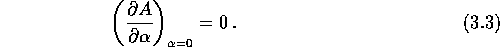The-dependence of the integral is contained inand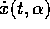, thusBy inserting equation (3.2) and integrating the second term by parts, we getThe integrated part vanishes due to the fixed end-points (boundary values). The condition for stationary values, equation (3.3), is therefore equal to the following relation: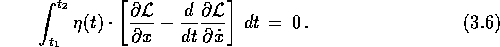In order to arrive at the equation of motion, the fundamental theorem of variational calculus is needed. It state that if the integral in equation (3.6) vanishes for everycontinuously differentiable in the interval, then the content of the brackets in the equation (3.6) must identically vanish in the same interval; that is, for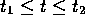. It therefore follows that A can have stationary values only if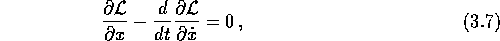which is the familiar Euler-Lagrange differential equation. By inserting the Lagrangian, one can then deduce the equation of motion for the mechanical system.

What is the point of going through this rather extensive deduction, just arrive at the well known Euler-Lagrange differential equation? In this case there are at least three motivations. Firstly, it shows that the Euler-Lagrange equations are in fact a result of the very compact Hamilton's principle. Secondly, it will be needed in the next section to justify the use of mixed boundary values. Thirdly, and most importantly, the principle is applied directly in the numerical implementation of the AVP.

The one-dimensional deduction presented in this section can easily be extended to any multidimensional case.Next: Equations of Motion Up: The Dynamics of Galaxies Previous: Simplifying Assumptions

Trond Hjorteland
Mon Jul 5 02:59:28 MET DST 1999